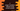# Swap two numbers without using a third number and using a macro in C# Introduction :

In this tutorial, we will swap two numbers using one macro and without using a third number. We are using the bitwise operator to swap these numbers.

With this program, you will learn how to swap two numbers without using a third number using a bitwise operator and using a macro.

## C program :

Before going into details, let’s check the program first :

``````#include <stdio.h>

//1
#define SWAP(a,b) a ^= b ^= a ^= b

int main(){
//2
int a,b;

//3
printf("Enter the first number to swap : ");
scanf("%d",&a);

//4
printf("Enter the second number : ");
scanf("%d",&b);

//5
SWAP(a,b);

//6
printf("First and second number after swap : %d and %d\n",a,b);

}
``````

### Explanation :

The commented numbers in the above program denote the step numbers below :

1. First, define one macro SWAP. This will take two numbers a and b and swap them using bitwise operator. a ^= b ^= a ^= b will swap a and b.
2. Define two integers a and b.
3. Ask the user to enter the first number. Enter it and store it in ‘a’.
4. Similarly, read and store it in ‘b’.
5. Now call the macro SWAP. Pass ‘a’ and ‘b’ to the macro. It will swap the numbers stored in the variables.
6. Finally, print out both numbers.

### Sample Output :

``````Enter the first number to swap : 3
Enter the second number : 2
First and second number after swap : 2 and 3

Enter the first number to swap : 23
Enter the second number : 33
First and second number after swap : 33 and 23

Enter the first number to swap : -23
Enter the second number : 567
First and second number after swap : 567 and -23
``````

### Conclusion :

This is the simplest method to swap two numbers in C. Instead of using a third variable, we can write just one line to swap two variables. You can also use one method and move the code to it. Try to run the program and drop one comment below if you have any queries.

You might also like :